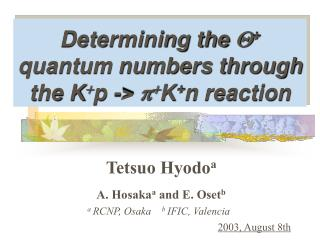Download PresentationDetermining the Q + quantum numbers through the K + p -> p + K + n reaction

# Determining the Q + quantum numbers through the K + p -> p + K + n reaction - PowerPoint PPT PresentationDownload Presentation## Determining the Q + quantum numbers through the K + p -> p + K + n reaction

- - - - - - - - - - - - - - - - - - - - - - - - - - - E N D - - - - - - - - - - - - - - - - - - - - - - - - - - -
##### Presentation Transcript

1. Determining the Q+ quantum numbers through the K+p -> p+K+n reaction Tetsuo Hyodoa A. Hosakaa and E. Osetb a RCNP, Osaka b IFIC, Valencia 2003, August 8th

2. Introduction Q+: 5-quark (4 quark + 1 anti-quark) LEPS, T. Nakano et al., Phys. Rev. Lett. 91 (2003) 012002 Quantum numbers are not yet determined Theory prediction Diakonov et al. (chiral quark soliton) Carlson et al. (quark model[QM]) Stancu et al. (QM + flavor-spin int.) Zhu (QCD sum rule) Capstick et al. (decay width analysis) : 1/2+, I=0 : 1/2-, I=0 : 1/2+, I=0 : 1/2-, I=0, 1, 2 : 1/2-, 3/2-, 5/2-, I=2 We study K+p -> p+K+n reaction to determine the quantum numbers ofQ+

3. A model for p-p -> K0pS E. Oset and M.J. Vicente Vacas, PLB386, 39(1996) Vertices are derived from the chiral Lagrangian Proportional to vanishes Dominant Assume finalp+is almost at rest

4. Diagrams Tree level (background) One loop

5. Possibilities of spin & parity 1/2-(KN s-wave resonance) 1/2+,3/2+(KN p-wave resonance) MR = 1540 MeV G = 20 MeV

6. Resonance term Amplitude of resonance term for K+p -> p+K+n :

7. Angular dependence

8. Mass distributions

9. Polarization test

10. Angular dependence : polarization test

11. Mass distributions : polarization test

12. Conclusions We calculate thep-p -> K0pSreaction using a chiral model, assuming the possible quantum numbers of Q+ baryon. • Resonance signal of the mass distribution • is always seen in the forward direction. • If we find the resonance with polarization • test, the quantum number of Q+ can be • determined as I=0, JP=1/2+ T. Hyodo, A. Hosaka, and E. Oset, nucl-th/0307105